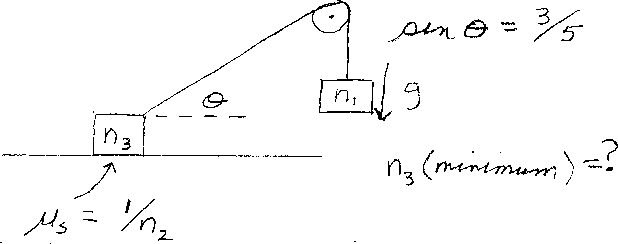Problem A9: In the figure above, the system is in static equilibrium. The coefficient of static friction between the block and the floor is &mu s = 1/n2. The mass of the block that is hanging down is n1 Kg. The tangent of the angle shown in the figure is tan &theta = 3/5. What is the minimum value of mass, n3 Kg, that the block on the table can have such that the system is in equilibrium?n1 = n2 = Input n3:
If you are currently in my class, you can record your grade by entering your name and student ID number (without the leading zeros) below and clicking on "record grade".
 First Name = Last Name = ID = Problem: